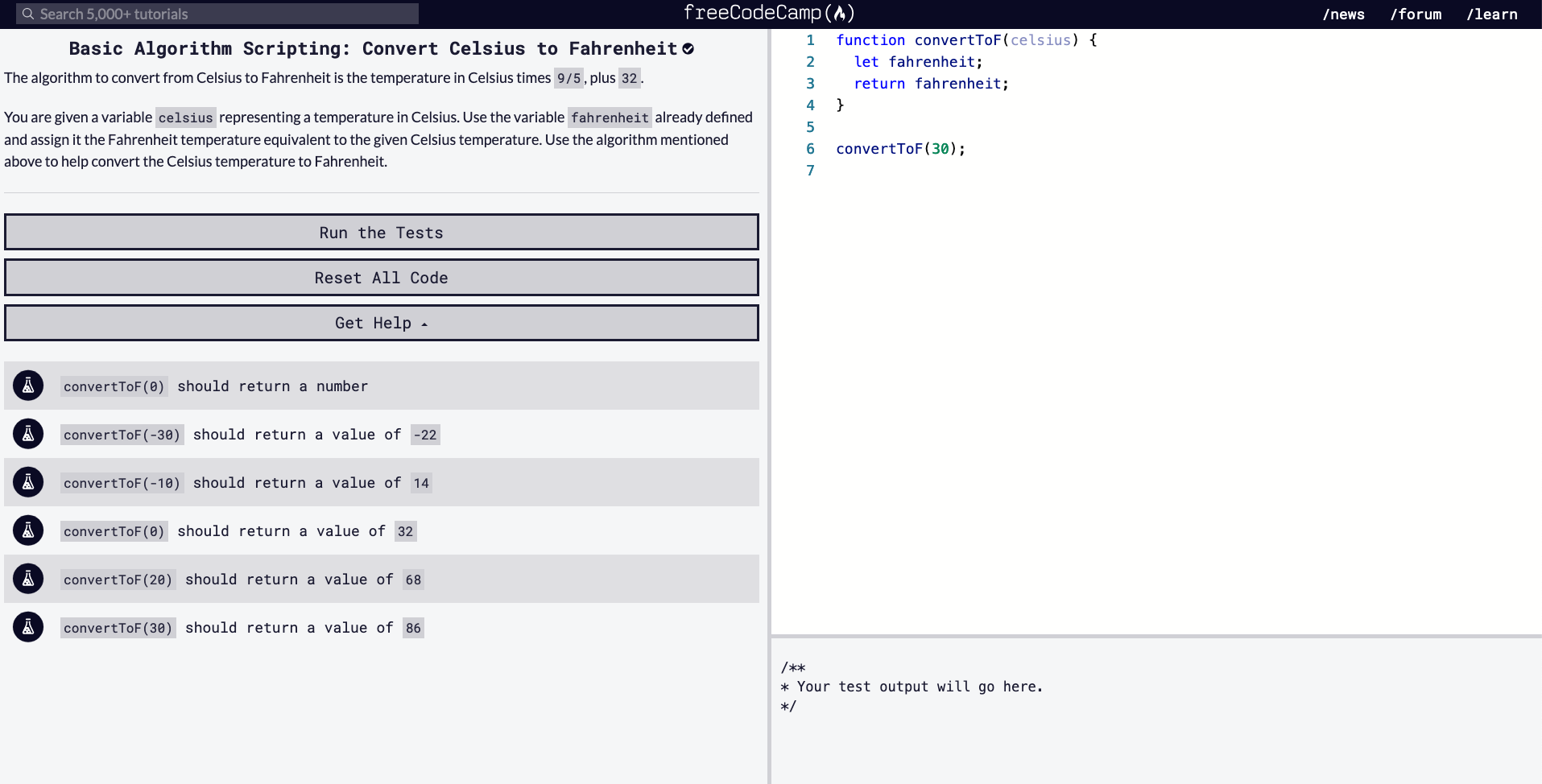# FCC Algorithm Challenges / Convert Celsius to Fahrenheit

### 4/20/2020``````function convertToF(celsius) {
let fahrenheit;
return fahrenheit;
}

convertToF(30);

// TESTS
// convertToF(0) should return a number
// convertToF(-30) should return a value of -22
// convertToF(-10) should return a value of 14
// convertToF(0) should return a value of 32
// convertToF(20) should return a value of 68
// convertToF(30) should return a value of 86
``````

Above is the starting code provided for Algorithm Scripting challenge, "Convert Celsius to Fahrenheit".

Our goal is to write a function that will take in a Celsius value (provided) and output the Fahrenheit value of that given temperature. Let's think this through. Here is how I would aim to solve this problem.

## Method

• Read the instructions first. Make sure you understand what it being asked of you.
• Read the starter code. Go line by line, just making sure you know what is going on initially.
• Have a look at the tests. If the problem isn't clear to you, looking at the tests might give you an inclination of what kind of output you should aim for (i.e. instead of returning an array, maybe the problem is only asking for an index within the array).
2. Think & Write

Now that you've read through the instructions, starter code, and tests, it's time to analyze what to do and in what order. It may be handy to write out pseudocode.

3. Code

Once you've thought about what you'd like to do, and in what order, start to convert your pseudocode into JavaScript code.

There's been too many times where I've tried to jump write into writing the code without thinking it through (in projects and coding challenges). That will leave you testing it way too many times, creating unnecessary variables, and running into more problems then you need to handle. If I try to follow the above method, it leaves me with a more clear mind of what I'm doing and hopefully writing some DRY code. Let's try to solve this problem now.

## Thoughts

• We're given the formula we need to convert Celsius to Fahrenheit in the instructions (Celsius * 9/5 + 32)
• The argument is the the Celsius temperature
• In the code, on line 2, they've provided a variable for us, `let fahrenheit;`
• Let us assign the conversion formula to the `fahrenheit` variable
• The next line (line 3) returns the `fahrenheit` variable so we know this function will return something

## Solution

**[SPOILER: SOLUTION TO CODE BELOW]** ```javascript function convertToF(celsius) { let fahrenheit = (celsius * 9 / 5) + 32; return fahrenheit; } ```

Alternative Solutions

``````// Without declaring a fahreinheit variable
function convertToF(celsius) {
return celsius * 9/5 + 32;
}
``````
``````// ES6 Solution, using an arrow function
const convertToF = celsius => celsius * 9/5 + 32;
``````

These solutions are little shorter and less verbose.